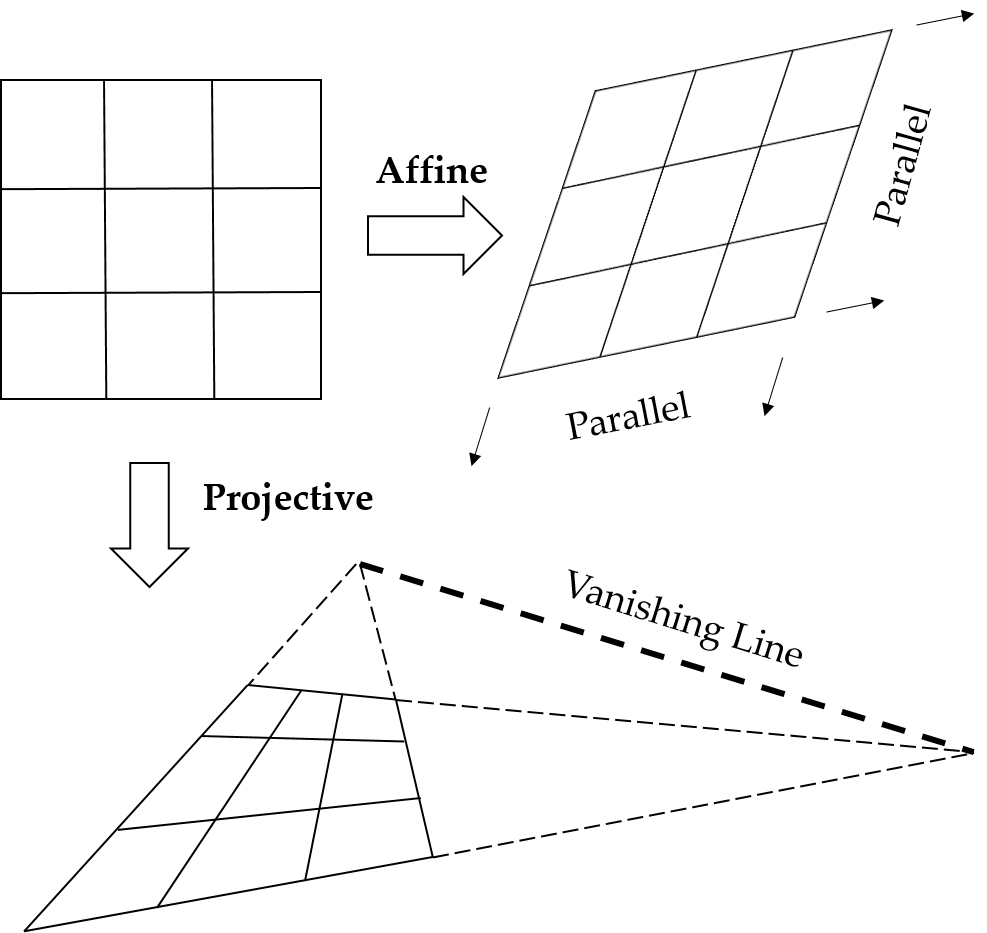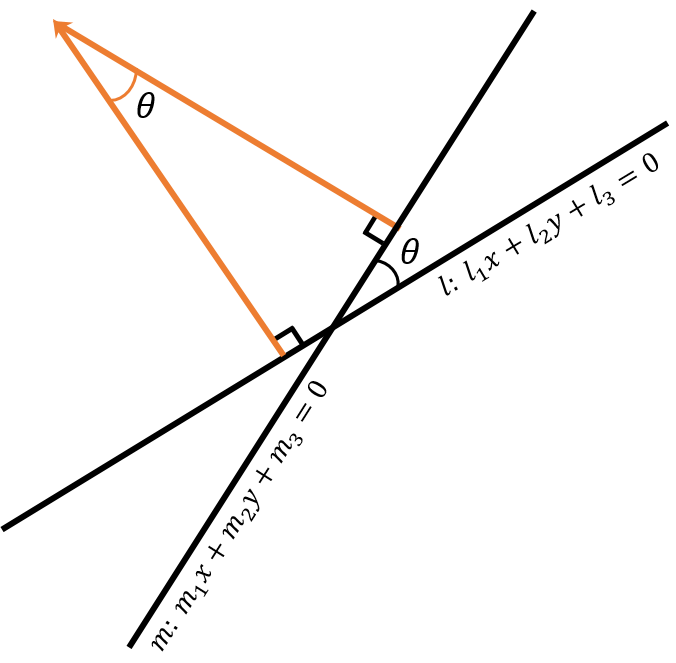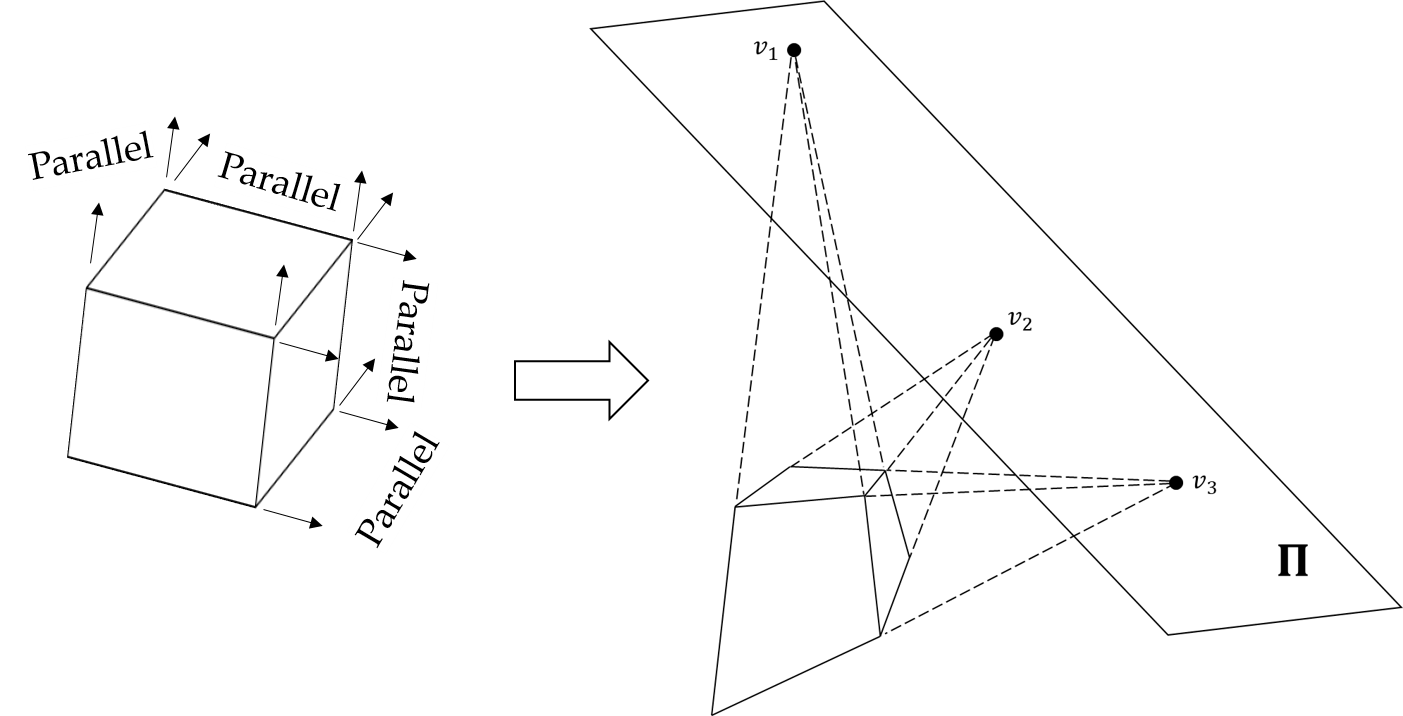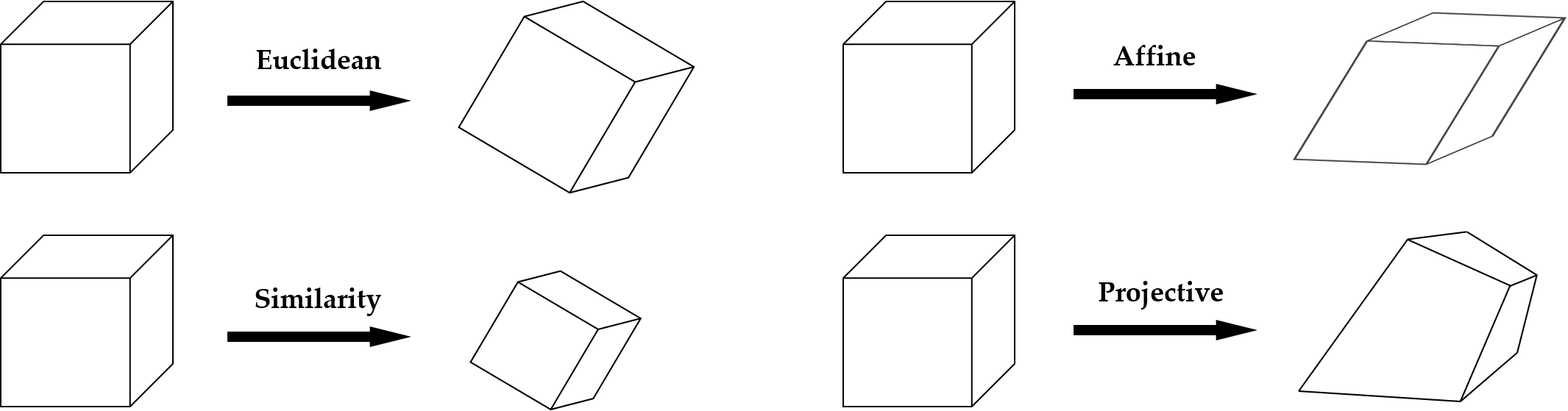# Hierarchy of Transformation

## 1. Affine and projectve transformations• For two arbitrary ideal points which are of the form $(x, y, 0)^t$, these points are always on the line $l_{\infty} = (0, 0, 1)^t$ since $(x_1, y_1, 0)^t x (x_2, y_2, 0)^t = l_{\infty}$.
• A point on $l_{\infty}$ is transformed to another point on $l_{\infty}$ by affine transformation. But it is transformed to a point on the vanishing line by projective transformation.

## 2. How to remove the projective distortion

• Projective transformation causes the distortion such that parallel lines in affine space are not parallel any more because a vanishing line comes about.
• Let the vanishing line be $l = (l_1, l_2, l_3)^t$, then $l$ is mapped to $l_{\infty}$ by a matrix $H$ as follows:
$\begin{equation} H = \left(\begin{array}{ccc} 1 & 0 & 0 \\ 0 & 1 & 1 \\ l_1 & l_2 & l_3 \end{array}\right) \end{equation}$
• While a point is transformed by $H$, a normal vector is transformed by $H^{-t}$. So $H^{-t}l = l_{\infty}$.
$\begin{equation} H^{-t}l = \left(\begin{array}{ccc} 1 & 0 & -\frac{l_1}{l_3} \\ 0 & 1 & -\frac{l_2}{l_3} \\ 0 & 0 & \frac{1}{l_3} \end{array}\right) \left(\begin{array}{c} l_1 \\ l_2 \\ l_3 \end{array}\right) = \left(\begin{array}{c} 0 \\ 0 \\ 1 \end{array}\right) = l_{\infty} \end{equation}$
• Therefore, $H^{-t}$ transforms any line $l$ into $l_{\infty}$, which means that it restores the projective distortion. But it still remains the affine distortion.

## 3. The angle between two lines• For two lines $l$ and $m$, the angle $\theta$ between them is the following:
\begin{aligned} cos\theta &= \frac{l_1 m_1 + l_2 m_2}{\sqrt{(l^2_1 +ㅣ^2_2)(m^2_1 +m^2_2)}} = \frac{l^t C^{*}_{\infty}m}{\sqrt{(l^t C^{*}_{\infty}l)(m^t C^{*}_{\infty}m)}} \\ \\ C^{*}_{\infty} &= \left(\begin{array}{ccc} 1 & 0 & 0 \\ 0 & 1 & 0 \\ 0 & 0 & 0 \end{array}\right) : \text{dual degenerate conic} \end{aligned}

## 4. How to remove the affine distortion

• Even though the projective distortion is removed, there is still the affine distortion, which a square shape turns into a parallelogram shape. In other words, the angle between lines is not preserved.
• Assume that two lines $l$, $m$, and dual degenerate conic $C^{*}_{\infty}$ in the original space are transformed to $l'$, $m'$ and $C'^{*}_{\infty}$ by affine transformation. Then $l' = H^{-t}l$, $m' = H^{-t}m$, and $C'^{*}_{\infty} = HC^{*}_{\infty}H^t$.
• For the angle $\theta$ between $l$ and $m$, the numerator of $cos\theta$ is $l^t C^{*}_{\infty} m = (l'^t H)(H^{-1}C'^{*}_{\infty} H^{-t})(H^t m') = l'^t C'^{*}_{\infty} m'$.
• When $l$ and $m$ are perpendicular, $l'^t C'^{*}_{\infty} m' = 0$. It yields as follows:
\begin{aligned} l'^t C'^{*}_{\infty} m' = l'^t H C^{*}_{\infty} H^t m' = (l'_1, l'_2, l'_3) \left(\begin{array}{cc} A & \overrightarrow{t} \\ \overrightarrow{0} & 1 \end{array}\right) \left(\begin{array}{cc} I & \overrightarrow{0} \\ \overrightarrow{0} & 0 \end{array}\right) \left(\begin{array}{cc} A & \overrightarrow{0} \\ \overrightarrow{t} & 1 \end{array}\right) \left(\begin{array}{c} m'_1 \\ m'_2 \\ m'_3 \end{array}\right) = 0 \\ \\ (l'_1, l'_2, l'_3) \left(\begin{array}{cc} AA^t & \overrightarrow{0} \\ \overrightarrow{0} & 0 \end{array}\right) \left(\begin{array}{c} m'_1 \\ m'_2 \\ m'_3 \end{array}\right) = (l'_1, l'_2) \left(\begin{array}{cc} s_{11} & s_{12} \\ s_{21} & s_{22} \end{array}\right) \left(\begin{array}{c} m'_1 \\ m'_2 \end{array}\right) = 0 \end{aligned}

where $\left(\begin{array}{cc} s_{11} & s_{12} \\ s_{21} & s_{22} \end{array}\right) = S = AA^t$.

• $S$ is symmetric, $s_{12} = s_{21}$, and $s_{22}$ can be set to $1$ since $S$ is defined up to scale.
• $s_{11} l'_1 m'_1 + s_{12} (l'_1 m'_2 +l'_2 m'_1) = 0$.
• If we get two pairs of $l$ and $m$ which are perpendicular, then $S$ can be calculated.
• Having calculated $S$, $H$ can be esimated assuming $A$ is positive-definite. From $S = AA^t$, the eigenvalues of $A$ are the positive square roots of teh eigenvalues of $S$.
• After removing the affine distortion, there still remains the similarity distortion.

## 5. Hierarchy of transformation in 3D is similar to that in 2D

• As a point on $l_{\infty}$ is transformed to another point on $l_{\infty}$ by affine transformation, a point on the plane $\Pi_{\infty} = (0, 0, 0, 1)^t$ is also transformed to another point on $\Pi_{\infty}$.
• As a point on $l_{\infty}$ is transformed to a point on the vanishing line by projective transformation, a point on $\Pi_{\infty} = (0, 0, 0, 1)^t$ is transformed to a point on the specific plane.• When a cube is transformed in projective space, each a pair of parallel lines is not parallel any more and intersects at a point which is not at infinity such as $v_1$, $v_2$, and $v_3$.
• These points $v_1$, $v_2$, and $v_3$ consist of a plane $\Pi$ which does correspond to $\Pi_{\infty}$ in affine space.
• If we know this plane $\Pi$, it can be transformed to $\Pi_{\infty}$ by some matrix $H$, so the projective distortion can be removed.
• Let $\Pi = (\pi_1, \pi_2, \pi_3, \pi_4)^t = (\overline{\pi}^t, \pi_4)^t$, then the following $H$ restores $\Pi$ to $\Pi_{\infty}$:
$\begin{equation} H = \left(\begin{array}{cc} A & \overrightarrow{0} \\ \overline{\pi}^t & \pi_4 \end{array}\right), H^{-1} = \left(\begin{array}{cc} A^{-1} & \overrightarrow{0} \\ -\frac{1}{\pi_4} \overline{\pi}^t A^{-1} & \frac{1}{\pi_4} \end{array}\right), H^{-t} = \left(\begin{array}{cc} A^{-t} & -\frac{1}{\pi_4} A^{-t} \overline{\pi} \\ \overrightarrow{0} & \frac{1}{\pi_4} \end{array}\right) \end{equation}$ $\begin{equation} H^{-t} \Pi = \left(\begin{array}{cc} A^{-t} & -\frac{1}{\pi_4} A^{-t} \overline{\pi} \\ \overrightarrow{0} & \frac{1}{\pi_4} \end{array}\right) \left(\begin{array}{c} \overline{\pi} \\ \pi_4 \end{array}\right) = \left(\begin{array}{c} \overrightarrow{0} \\ 1 \end{array}\right) = \Pi_{\infty} \end{equation}$
• $\overline{\pi}$ is the orientation of $\Pi$ and $\Pi$ is a homogeneous representation, so $\Pi \sim \frac{1}{\pi_4} \Pi$.## Reference

 Hartley, R. and Zisserman, A. (2003) Multiple View Geometry in Computer Vision. 2nd Edition, Cambridge University Press, Cambridge.